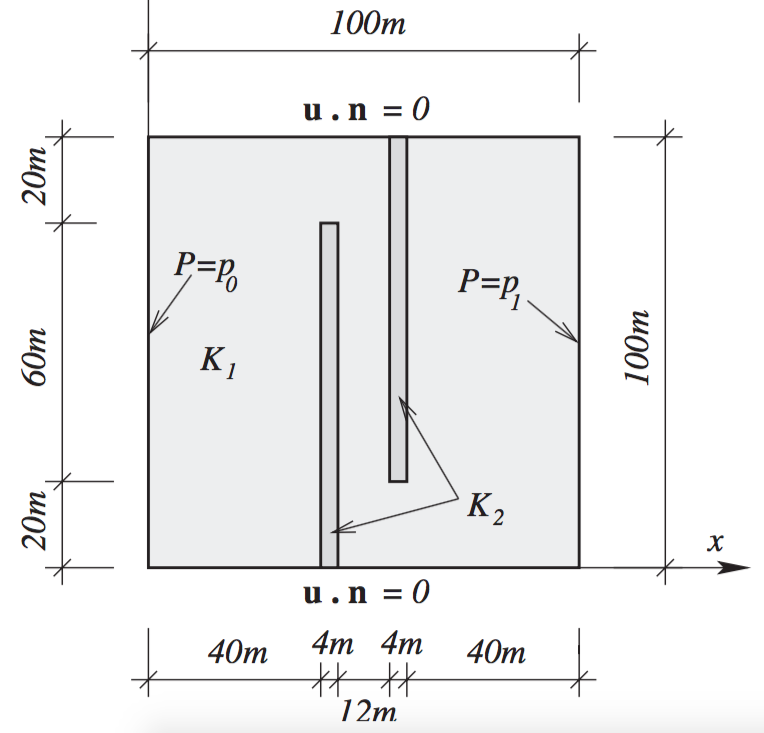# Darcy flow through a channel

This example can be found from [CL07].

## 1. Running the case

The command line to run this case is :

``mpirun -np 4 feelpp_toolbox_mixed-poisson-model --case "github:{repo:toolbox,path:examples/modules/poisson/examples/2Dchannel}"``
Case option
`--case "github:{repo:toolbox,path:examples/modules/poisson/examples/2Dchannel}"`

## 2. Input parameters

Notation Quantity Type Unit

$\kappa_1$

Permeability of $\Omega_1$

scalar

$m^2Pa^{-1}s^{-1}$

$\kappa_2$

Permeability of $\Omega_2$

idem

idem

## 3. Model & toolbox

We consider a $100\times100$ square channeled with two poorly porous panes.The top and bottom boundaries $\Gamma_{wall}$ are labelled `wall`, the left inlet $\Gamma_{left}$ is `left` and the right outlet $\Gamma_{right}$ is `right`. The main porous domain is `omega1` while the panes are labeled `omega2`. The interface is labeled `interface`.

This example runs within the `mixed-poisson` toolbox.

## 4. Boundary conditions

We impose a Neumann no-flow condition on the `wall` and Dirichlet conditions on the inlet and outlet :

$\left\{\begin{array}{ll} p=1&\text{on }\Gamma_{left}\\ p=0&\text{on }\Gamma_{right}\\ \underline u\cdot\underline n=0&\text{on }\Gamma_{wall} \end{array}\right.$

## 7. Reference

[CL07] M. R. Correa, A. F. D. Loula, Stabilized velocity post-processings for Darcy flow in heterogeneous porous media, Commun. Numer. Meth. Engng 2007; 23:461–489